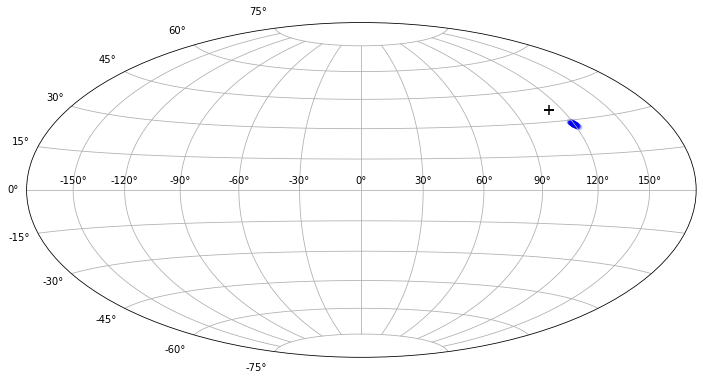# Galactic backtracking

The following setup shows how to use CRPropa for backtracking simulations.
In the JF12 model the Galaxy is a sphere of 20 kpc radius. For the magnetic field we are going to consider the regular component of the JF2012 model. The large-scale (striated) and small-scale (turbulent) random components can optionally be activated with the outcommented sections and a random seed can be set for reproducability.
:

from crpropa import *

# magnetic field setup
B = JF12Field()
#seed = 691342
#B.randomStriated(seed)
#B.randomTurbulent(seed)

# simulation setup
sim = ModuleList()
sim.add(PropagationCK(B, 1e-4, 0.1 * parsec, 100 * parsec))
obs = Observer()
obs.add(ObserverSurface( Sphere(Vector3d(0), 20 * kpc) ))
# obs.onDetection(TextOutput('galactic_backtracking.txt', Output.Event3D))
print(sim)

ModuleList
Propagation in magnetic fields using the Cash-Karp method. Target error: 0.0001, Minimum Step: 0.0001 kpc, Maximum Step: 0.1 kpc
Observer
ObserverSurface: << Sphere:
Center: 0 0 0

Flag: '' -> ''
MakeInactive: yes



## Backtracking a single cosmic ray

Let’s assume we observed a 10 EeV cosmic ray coming from the direction given by longitude and colatitude (1.95, 0.96) radian and want to investigate its direction before having traversed the Galaxy.

Backtracking corresponds to forward-tracking a particle of the opposite charge, thus we select an anti-proton, which in the HEP ID numbering scheme is denoted by a negative sign. Assuming the cosmic ray was a proton the backtracking turns out as follows.

:

pid = - nucleusId(1,1)  # (anti-)proton
energy = 10 * EeV
position = Vector3d(-8.5, 0, 0) * kpc
lat = 0.96
lon = 1.95
direction = Vector3d()
direction.setRThetaPhi(1, lat, lon)
p = ParticleState(pid, energy, position, direction)
c = Candidate(p)

sim.run(c)
print(c)

d1 = c.current.getDirection()  # direction at Galactic border
print('Galactic deflection %.2f radian' % direction.getAngleTo(d1))

CosmicRay at z = 0
source:  Particle -1000010010, E = 10 EeV, x = -0.0085 0 0 Mpc, p = -0.303249 0.760996 0.57352
current: Particle -1000010010, E = 10 EeV, x = -0.0144674 0.011531 0.00759822 Mpc, p = -0.434112 0.764527 0.476493


## Backtracking including uncertainties

The impact of the cosmic ray uncertainties on backtracked directions can be investigated with a MC approach. In the following, the cosmic ray energy and direction are varied within the statistical uncertainties before backtracking.

:

R = Random()  # CRPropa random number generator

pid = - nucleusId(1,1)
meanEnergy = 10 * EeV
sigmaEnergy = 0.1 * meanEnergy  # 10% energy uncertainty
position = Vector3d(-8.5, 0, 0) * kpc
lat0 = 0.96
lon0 = 1.95
meanDir = Vector3d()
meanDir.setRThetaPhi(1, lat0, lon0)
sigmaDir = 0.002  # 1 degree directional uncertainty

lons, lats = [], []
for i in range(100):
energy = R.randNorm(meanEnergy, sigmaEnergy)

c = Candidate(ParticleState(pid, energy, position, direction))
sim.run(c)

d1 = c.current.getDirection()
lons.append(d1.getPhi())
lats.append(d1.getTheta())


## (Optional) Plotting

Finally we are plotting a skymap of the observed direction along with the distribution of directions at the galactic border.

:

%matplotlib inline
import matplotlib.pyplot as plt
import numpy as np

# Angle definitions:
# CRPropa uses
#   longitude (phi) [-pi, pi] with 0 pointing in x-direction
#   colatitude (theta) [0, pi] with 0 pointing in z-direction
# matplotlib expects
#   longitude [-pi, pi] with 0 = 0 degrees
#   latitude [pi/2, -pi/2] with pi/2 = 90 degrees (north)
lat0 = np.pi/2 - lat0
lats = np.pi/2 - np.array(lats)

plt.figure(figsize=(12,7))
plt.subplot(111, projection = 'hammer')
plt.scatter(lon0, lat0, marker='+', c='black', s=100)
plt.scatter(lons, lats, marker='o', c='blue', linewidths=0, alpha=0.2)
plt.grid(True)## Backtracking to Generate a Lens

The following is an example for a backtracking simulation with a uniform isotropic coverage suitable to generate a magnetic lens. Here, anti-particles are emitted following the healpic scheme to achieve an uniform coverage of the starting direction. Please note that for production use, nside = 1024 should be used and as well a fine binning of rigidities extendig down to ~0.1 EeV is required, e.g. $$10^{16.99}$$ eV; $$10^{17.01}$$ eV; $$10^{17.03}$$ eV … ;. The backtracking data can be post processed with the create-lens.py program.

:

from crpropa import *
import healpy

def backtrackingRun(logE=18., nside=16):
"""Galactic Lens: Backtracking run

Runs the backtracking simulation for a given rigidity and
healpy pixel configuration. Creates a file with detected
candidate properties at r_gal=20*kpc.

Input
-----
logE=18. : float
log10(R/V), rigidity of the backtracked particles

nside=16 : int
healpix parameter, should be increased to ~1024 for production
of real lenses

Returns
-------
"""

# magnetic field setup
B = JF12Field()
seed = 1703202123
B.randomStriated(seed)
B.randomTurbulent(seed)

# simulation setup
sim = ModuleList()
sim.add(PropagationCK(B, 1e-4, 0.1 * parsec, 100 * parsec))
obs = Observer()
obs.add(ObserverSurface( Sphere(Vector3d(0.), 20 * kpc) ))

ofname = 'galactic_backtracking_{:.2f}.h5'.format(logE)
print("Writing output to {}".format(ofname))
out = HDF5Output(ofname, Output.Event3D)
obs.onDetection(out)

pid = - nucleusId(1,1)  # (anti-)proton
energy = 10**logE * electronvolt

print("Running at 10**{} eV = {} EeV".format(logE, energy / EeV))

position = Vector3d(-8.5, 0, 0) * kpc

# submit a particle in every direction of a healpix map, 256 per pixel of the
# lens
nparts = healpy.nside2npix(nside)
print('simulating {} particles'.format(nparts))
# Use candidate vector to enable multi core processing
cv = CandidateVector()
print("Preparing Particles ...")
for i in range(nparts):
v = healpy.pix2vec(nside, i)
direction = Vector3d(v, v, v)
p = ParticleState(pid, energy, position, direction)
c = CandidateRefPtr(Candidate(p))
cv.push_back(c)

sim.setShowProgress()
sim.run(cv)
out.close()
print ("Finished!")

:

backtrackingRun()

Writing output to galactic_backtracking_18.00.h5
Running at 10**18.0 eV = 1.0 EeV
simulating 3072 particles
Preparing Particles ...